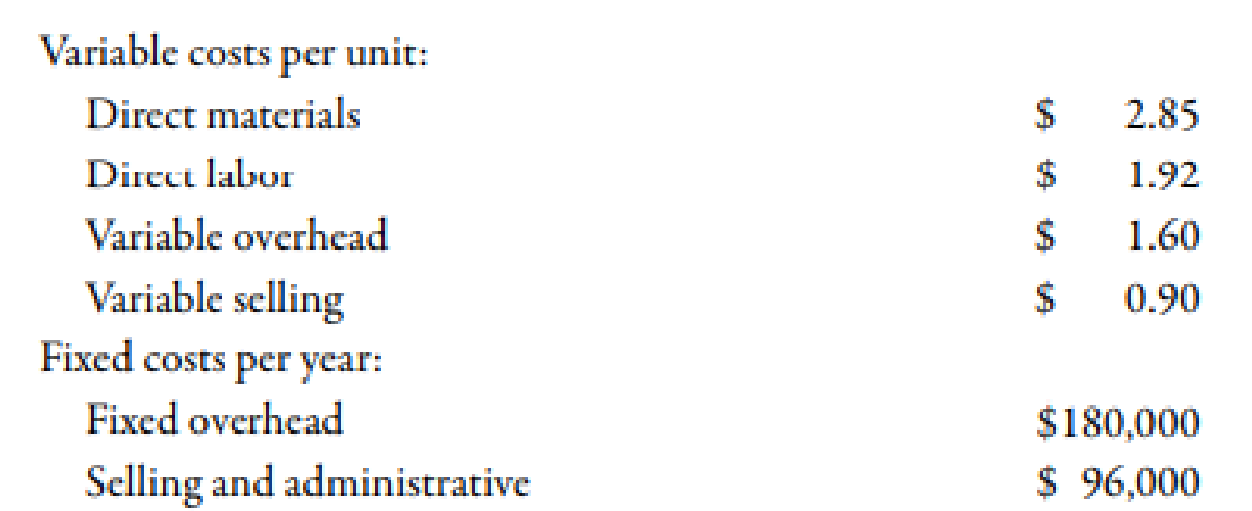Chapter 3, Problem 66P### Managerial Accounting: The Corners...

7th Edition
Maryanne M. Mowen + 2 others
ISBN: 9781337115773

#### Solutions

Chapter
Section### Managerial Accounting: The Corners...

7th Edition
Maryanne M. Mowen + 2 others
ISBN: 9781337115773
Textbook Problem
120 views

# Variable-Costing and Absorption-Costing IncomeBorques Company produces and sells wooden pallets that are used for moving and stacking materials. The operating costs for the past year were as follows:During the year, Borques produced 200,000 wooden pallets and sold 204,300 at $9 each. Borques had 8,200 pallets in beginning finished goods inventory; costs have not changed from last year to this year. An actual costing system is used for product costing.Required: 1. What is the per-unit inventory cost that is acceptable for reporting on Borques’s balance sheet at the end of the year ? How many units are in ending inventory? What is the total cost of ending inventory? 2. Calculate absorption-costing operating income. 3. CONCEPTUAL CONNECTION What would the per-unit inventory cost be under variable costing? Does this differ from the unit cost computed in Requirement 1? Why? 4. Calculate variable-costing operating income. 5. Suppose that Borques Company had sold 196,700 pallets during the year. What would absorption-costing operating income have been? Variable-costing operating income? 1. To determine Calculate per unit cost of inventory. Also, calculate the units of ending inventory and total cost of ending inventory. Explanation Cost: Cost can be defined as the cash and cash equivalent which is incurred against the products or its related services which will benefit the organization in the future. There are two types of costs that are fixed and variable costs. Calculation of per unit cost of inventory:  Particulars Amount ($) Direct material 2.85 Direct labor 1.92 Variable overhead 1.60 Fixed overhead1 0.90 Total 7.27

Table (1)

Therefore, per unit cost of inventory is \$7.27.

Use the following formula to calculate the units of ending inventory.

Units of Ending Inventory=(Opening Finished Goods+Manufactured GoodsClosing Finished Goods)

Substitute 8,200 units for opening finished goods, and 200,000 units for manufactured goods and 204,300 units for closing finished goods in the above formula.

Units of Ending Inventory=(8,200 units+200,000 units204,300units)=3,900 units

Therefore, a unit of ending inventory is 3,900 units

2.

To determine

Compute the operating income with the help of absorption costing.

3.

To determine

Calculate per unit cost of inventory with the help of variable costing. Also, identify the difference in the amount in part 1.

4.

To determine

Compute the operating income with the help of variable costing.

5.

To determine

Compute the operating income with the help absorption costing and variable costing:

### Still sussing out bartleby?

Check out a sample textbook solution.

See a sample solution

#### The Solution to Your Study Problems

Bartleby provides explanations to thousands of textbook problems written by our experts, many with advanced degrees!

Get Started